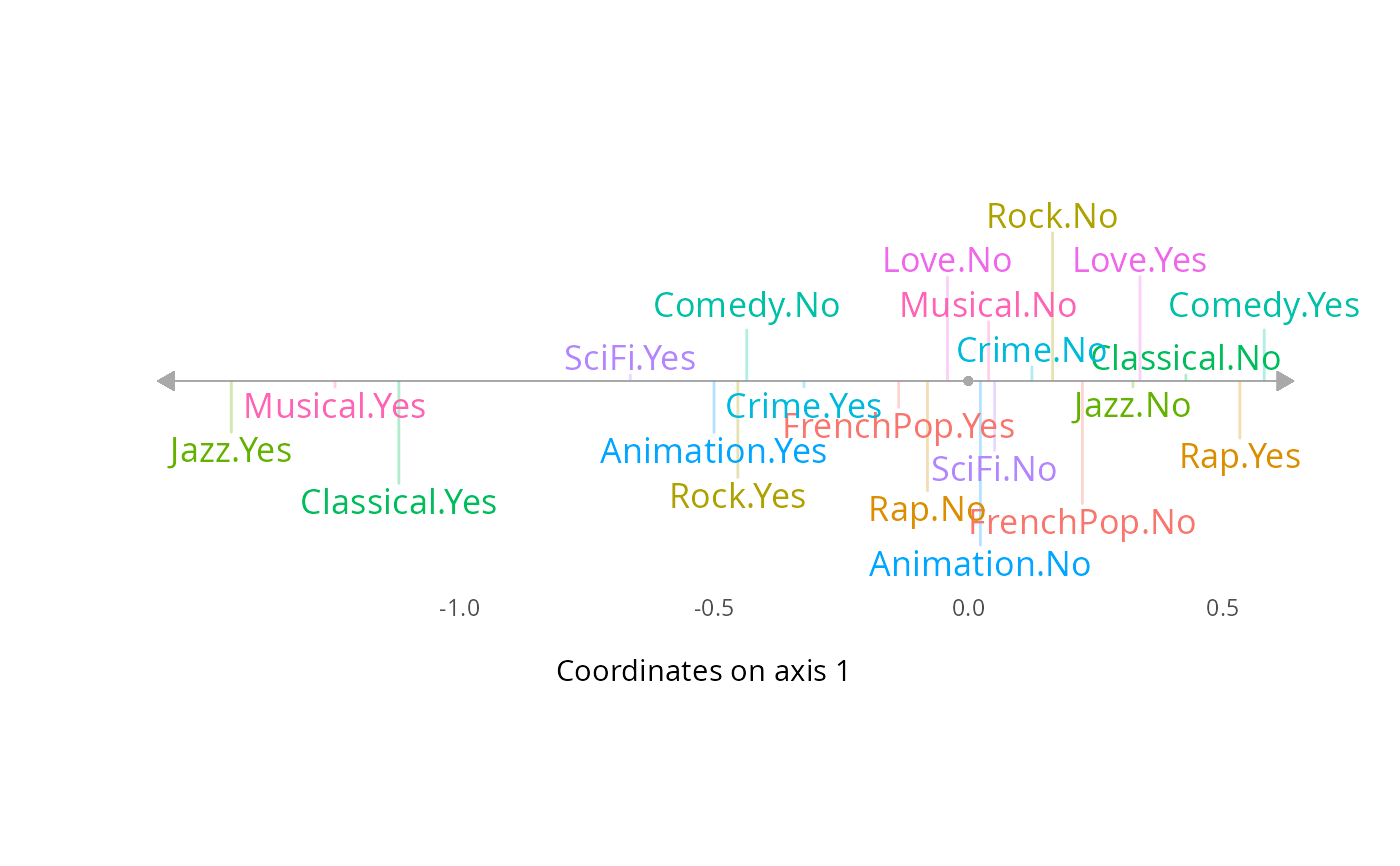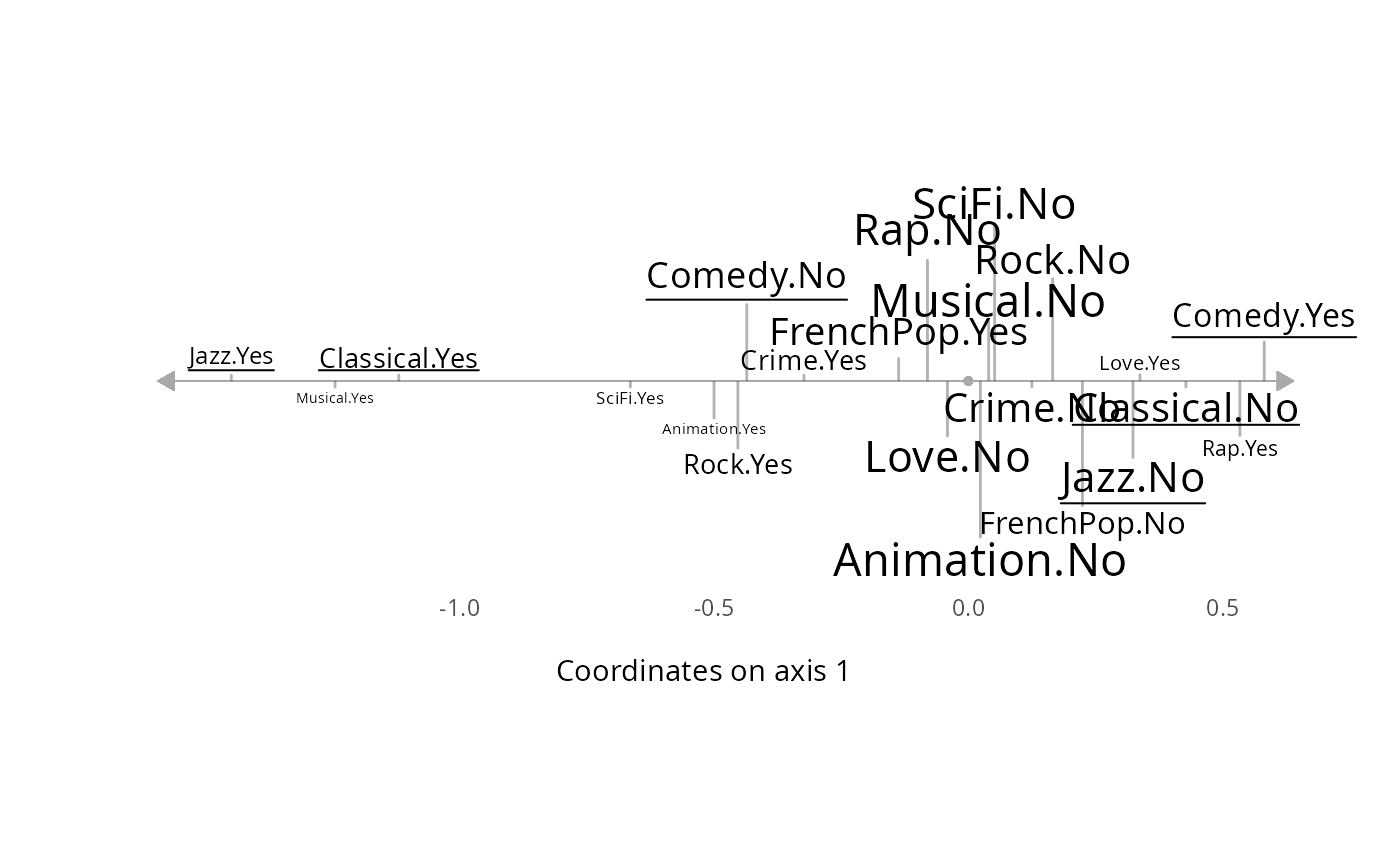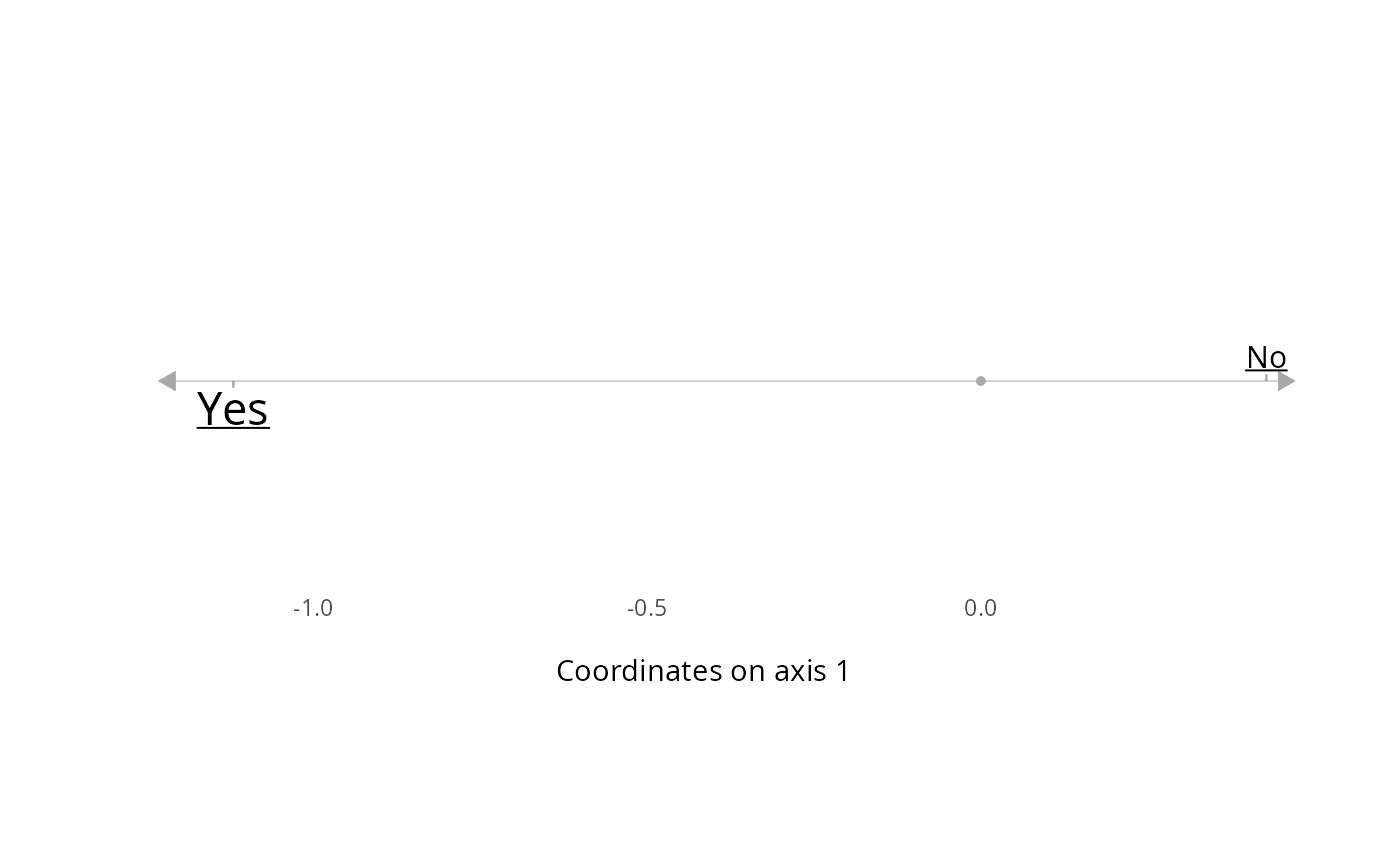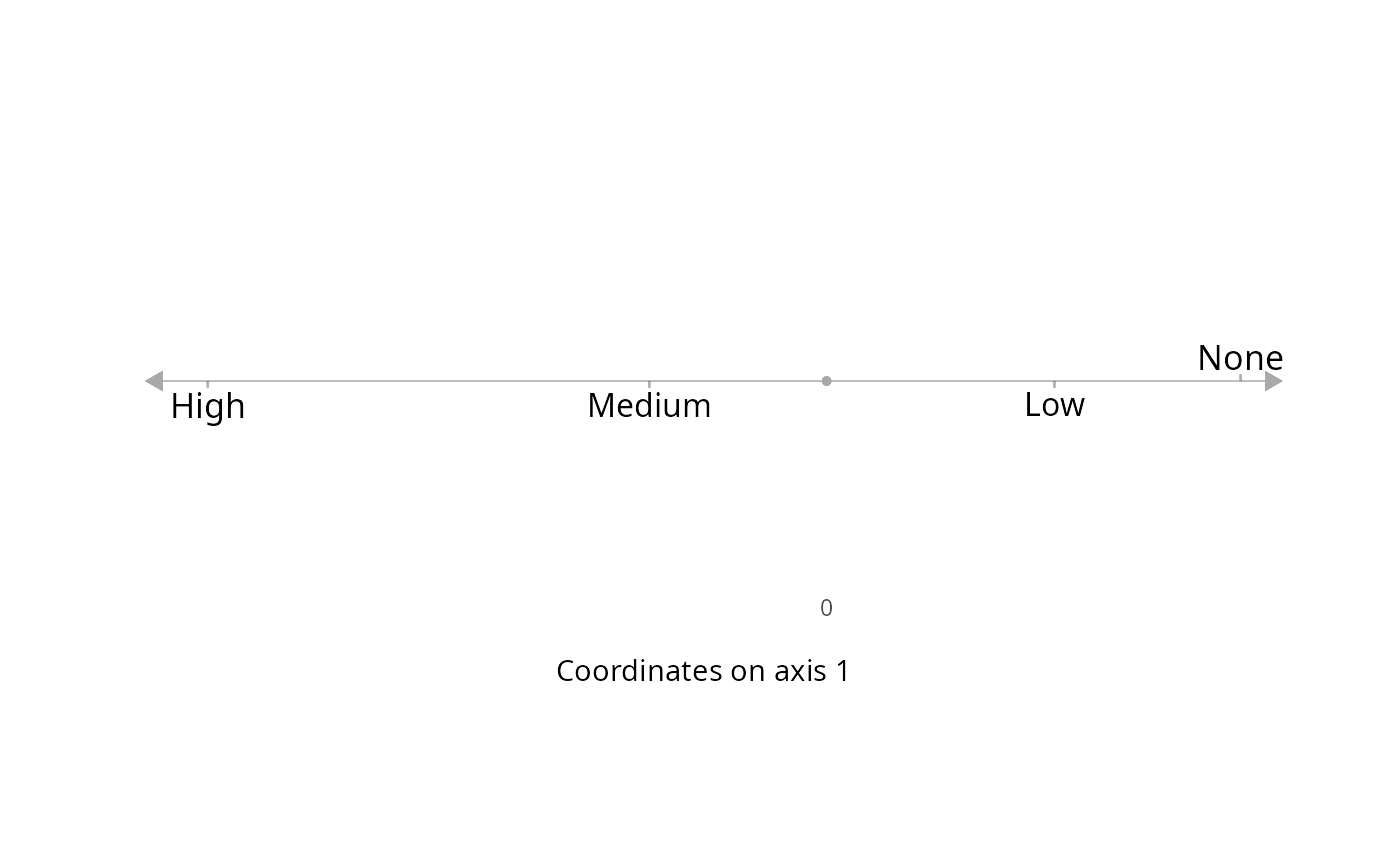Plots variables on a single axis of a Multiple Correspondence Analysis. Variables can be active or supplementary.

ggaxis_variables(resmca, var = NULL, axis = 1, prop = NULL,
underline = FALSE, col = NULL)

## Arguments

resmca

object of class MCA, speMCA, csMCA, stMCA or multiMCA.

var

If NULL (default), all the active variables of the MCA are plotted. If a character string, the named active variables of the MCA is plotted. If a factor, it is plotted as a supplementary variable.

axis

numeric value. The MCA axis to plot. Default is 1.

prop

If NULL (default), the size of the labels is constant. If "freq", the size is proportional to the weights of categories. If "ctr", it's proportional to the contributions of categories (only used for active variables). If "cos2", it's proportional to the squared cosines of the categories. If "pval", it's proportional to 1 minus the p-values of typicality tests (only used for supplementary variables). If "cor", it's proportional to the point biserial correlation of the categories (only used for supplementary variables).

underline

logical. If TRUE, the labels of the categories with contributions above average are underlined. Default is FALSE. Only used for active variables.

col

character string. Color name for the labels of the categories. If NULL and var=NULL, the default palette of ggplot2 is used, with one color per variable. If NULL and var is not NULL, labels are black.

## Value

a ggplot2 object

## Author

Nicolas Robette

ggcloud_variables

## Examples

# specific MCA of Taste example data set
data(Taste)
junk <- c("FrenchPop.NA", "Rap.NA", "Rock.NA", "Jazz.NA", "Classical.NA",
"Comedy.NA", "Crime.NA", "Animation.NA", "SciFi.NA", "Love.NA",
"Musical.NA")
mca <- speMCA(Taste[,1:11], excl = junk)
# plots all the active categories on axis 1
ggaxis_variables(mca)# the same with other plotting options
ggaxis_variables(mca, prop = "freq", underline = TRUE, col = "black")# plots Active variable Classical on axis 1
ggaxis_variables(mca, var = "Classical", axis = 1, prop = "ctr", underline = TRUE)# plots supplementary variable Educ on axis 1
ggaxis_variables(mca, var = Taste\$Educ, axis = 1, prop = "pval")